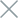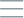• Home
• Tags
• Series
•# Evolutionary Search Algorithm in PHP: Explained with Example

The Evolutionary Search Algorithm is a powerful method in PHP programming, inspired by the natural process of evolution. It is an optimization approach based on reproductive mechanisms and natural selection to find the best solutions within a search space.

## How the Evolutionary Search Algorithm Works

The Evolutionary Search Algorithm begins by generating a set of initial "individuals," representing potential solutions. It then uses operators like crossover and mutation to create new generations of individuals. The fitness of individuals in each generation is evaluated using an assessment function to determine the quality of the solution.

• Natural Optimization Approach: This algorithm draws inspiration from natural evolution to seek the best solutions.
• Blend of Exploration and Optimization: The Evolutionary Search Algorithm combines the ability to explore new solutions and optimize existing ones.

• Parameter Consideration Required: For effective functioning, the algorithm demands careful tuning and selection of parameters such as crossover and mutation rates.

## Example and Explanation

Consider an example of using the Evolutionary Search Algorithm to find the maximum contiguous sum of a subarray within an integer array using PHP.

``````function evolutionarySearch(\$array, \$numGenerations) {
\$populationSize = count(\$array);
\$population = \$array;

for (\$generation = 0; \$generation < \$numGenerations; \$generation++) {
\$population = evolvePopulation(\$population);
}

return max(\$population);
}

function evolvePopulation(\$population) {
// Crossover and mutation operations here
// Return a new population
}

\$array = array(1, -2, 3, 4, -5, 6);
\$numGenerations = 50;

\$maxSum = evolutionarySearch(\$array, \$numGenerations);
echo "Maximum contiguous sum found: \$maxSum";
``````

In this example, we use the Evolutionary Search Algorithm to find the maximum contiguous sum of a subarray within an integer array. Each individual in the initial population represents a subarray of the array. Through generations of evolution, we use operations like crossover and mutation to create new generations of the population. The result is the maximum contiguous sum found through the optimization process.

While this example demonstrates how the Evolutionary Search Algorithm can be used to optimize a subarray within an array, it can also be applied to other optimization problems in PHP.